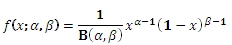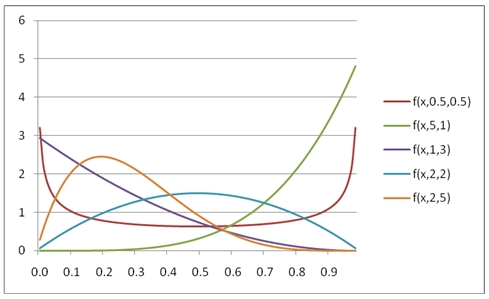# SQL Server BETA.DIST function

BETAPDF

Updated: 31 July 2010

Use BETAPDF to calculate the probability density function for the beta distribution.

The probability density function of the beta distribution is:Syntax
SELECT [wctStatistics].[wct].[BETAPDF] (
<@X, float,>
,<@P, float,>
,<@Q, float,>
,<@A, float,>
,<@B, float,>)
Arguments
@X
is any real number. @X is an expression of type float or of a type that implicitly converts to float@X is the value between @P and @Q at which to evaluate the function.
@P
is any real number. @P is an expression of type float or of a type that implicitly converts to float@A is a parameter to the distribution.
@Q
is any real number. @Q is an expression of type float or of a type that implicitly converts to float@Q is a parameter to the distribution.
@A
is any real number. @A is an expression of type float or of a type that implicitly converts to float@A is the lower bound to the distribution. If you enter NULL, @A defaults to zero (0).
@B
is any real number. @B is an expression of type float or of a type that implicitly converts to float.   @B is the upper bound to the distribution. If you enter NULL, @B defaults to one (1).

Return Types
float
Remarks
·         If @X ≤ 0, BETAPDF will return an error.
·         If @P ≤ 0 or @Q ≤ 0, BETAPDF will return an error
·         If @X< @A or @X > @B or @A = @B, BETAPDF will return an error.
·         If you enter NULL for @A and @B, BETAPDF will use zero and one.
·         For the cumulative density function, use BETADIST.
Examples
SELECT wct.BETAPDF(7,5,10,6,8)
This produces the following result
----------------------
0.610961914062504

(1 row(s) affected)

You can use the SeriesFloat function from the XLeratorDB/math library to generate a dataset which can be pasted into EXCEL to generate a graph of the function.
SELECT SeriesValue
,wct.BETAPDF(SeriesValue, 0.5, 0.5, NULL, NULL) as [f(x,0.5,0.5)]
,wct.BETAPDF(SeriesValue, 5, 1, NULL, NULL) as [f(x,5,1)]
,wct.BETAPDF(SeriesValue, 1, 3, NULL, NULL) as [f(x,1,3)]
,wct.BETAPDF(SeriesValue, 2, 2, NULL, NULL) as [f(x,2,2)]
,wct.BETAPDF(SeriesValue, 2, 5, NULL, NULL) as [f(x,2,5)]
FROM wct.SeriesFloat(.01, .99, .01,NULL,NULL)
This is an EXCEL-generated graph of the results:### SupportCopyright 2008-2021 Westclintech LLC         Privacy Policy        Terms of Service